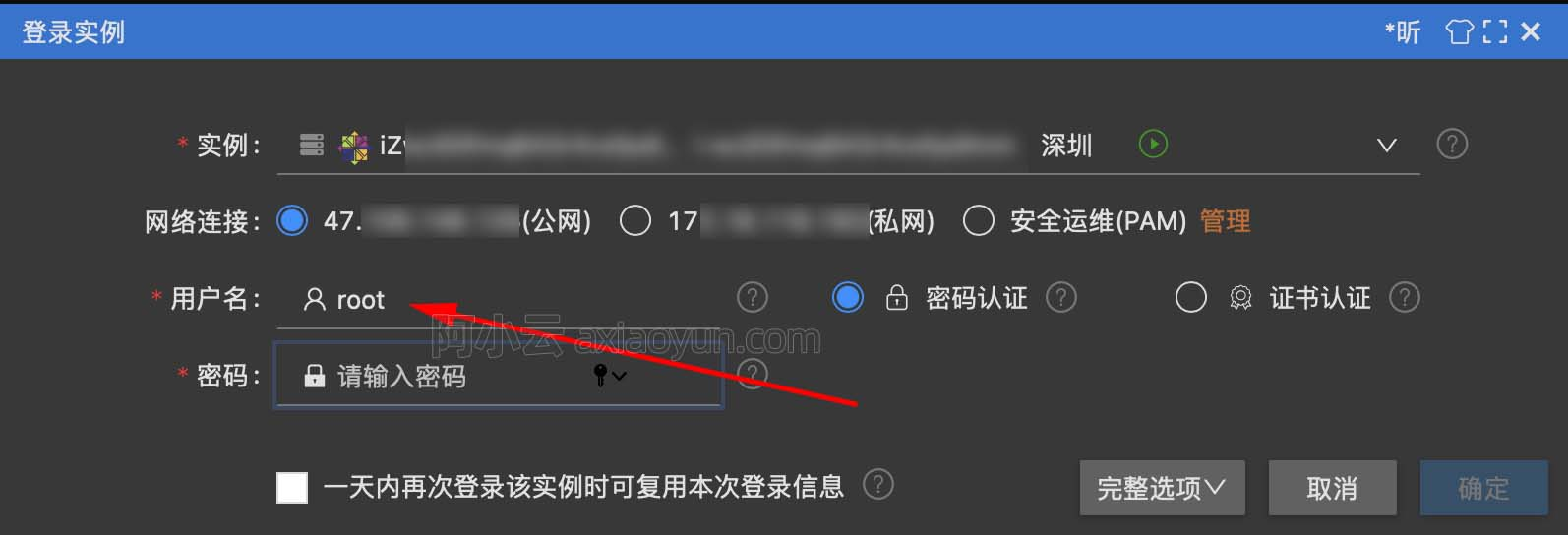# php设计模式总结-工厂模式

+关注继续查看

class DbFactory
{

function static factory($db_class_name) {$db_class_name = strtolower($db_class_name); if (include_once 'Drivers/' .$db_class_name . '.php') {

$classname = 'Driver_' .$db_class_name;
return new $db_class_name; } else { throw new Exception ('对应的数据库类没找到'); } } } DbFactory::factory("mysql"); DbFactory::factory("oracle"); 在thinkphp框架中也有对应的实现： Db.class.php就是一个工厂类(也可以叫做数据库中间层，之所以叫做中间层，是因为可以操作mysql、oracle等各数据库。而这个类就是中间层作用,屏蔽掉具体的实现。让程序员可以不改动原来的查询代码。中间层来对接mysql、oracle等数据库。 Db.class.php中有个factory()方法来创建不同的数据库实例 public function factory($db_config='') {
// 读取数据库配置
$db_config =$this->parseConfig($db_config); if(empty($db_config['dbms']))
throw_exception(L('_NO_DB_CONFIG_'));
// 数据库类型
$this->dbType = ucwords(strtolower($db_config['dbms']));
$class = 'Db'.$this->dbType;
if(is_file(CORE_PATH.'Driver/Db/'.$class.'.class.php')) { // 内置驱动$path = CORE_PATH;
}else{ // 扩展驱动
$path = EXTEND_PATH; } // 检查驱动类 if(require_cache($path.'Driver/Db/'.$class.'.class.php')) {$db = new $class($db_config);
// 获取当前的数据库类型
if( 'pdo' != strtolower($db_config['dbms']) )$db->dbType = strtoupper($this->dbType); else$db->dbType = $this->_getDsnType($db_config['dsn']);
if(APP_DEBUG)  $db->debug = true; }else { // 类没有定义 throw_exception(L('_NOT_SUPPORT_DB_').': ' .$db_config['dbms']);
}
return \$db;
}

UserFactory 用户工厂类，负责生成不同的用户类
User：用户类的基类，所有用户类都是继承这个类9388 0Xshell使用SSH远程登录阿里云ECS服务器CentOS7
10179 07746 0windows server 2008阿里云ECS服务器安全设置

5454 09423 03827 016804 046844 03227 01129 0
wangtaotao

167

0

《SaaS模式云原生数据仓库应用场景实践》

《看见新力量：二》电子书